# SAT Math Multiple Choice Question 51: Answer and Explanation

### Test Information

Question: 51

1.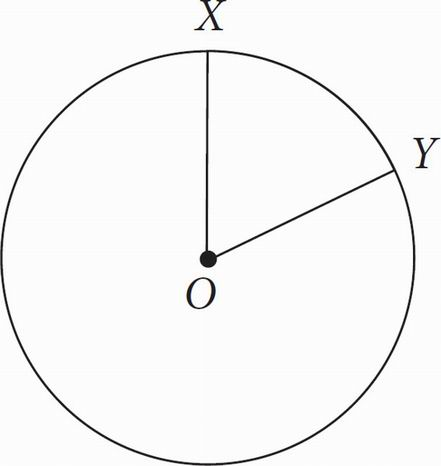In the figure above, circle O has a radius of 8, and angle XOY measures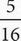π radians. What is the measure of minor arc XY ?

• A.π
• B.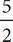π
• C. 5π
• D. 16π

B Because the question wants arc length and gives you the measure of the central angle in radians, you can use the formula s = to find the arc length: s = (8)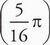=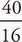π, which reduces to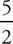π, which is (B).